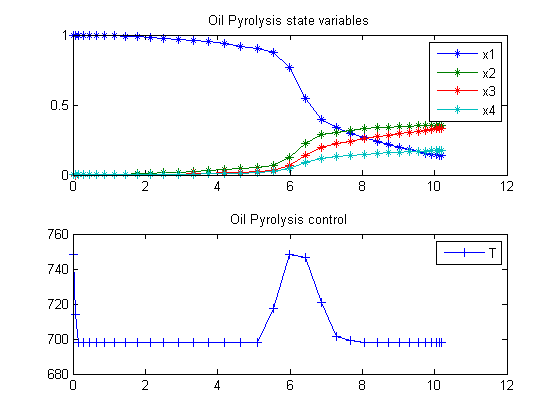75  Oil Shale Pyrolysis

Dynamic Optimization of Batch Reactors Using Adaptive Stochastic Algorithms 1997, Eugenio F. Carrasco, Julio R. Banga

Case Study II: Oil Shale Pyrolysis

75.1  Problem description

A very challenging optimal control problem is the oil shale pyrolysis case study, as considered by Luus (1994). The system consists of a series of five chemical reactions:

A1 -> A2

A2 -> A3

A1+A2 -> A2+A2

A1+A2 -> A3+A2

A1+A2 -> A4+A2

This system is described by the differential equations

 dx1 dt
= −k1*x1−(k3+k4+k5)*x1*x2
 dx2 dt
= k1*x1k2*x2+k3*x1*x2
 dx3 dt
= k2*x2+k4*x1*x2
 dx4 dt
= k5*x1*x2

where the state variables are the concentrations of species, Ai, i = 1, ..., 4. The initial condition is

 x(t0) = [1  0  0  0]′

The rate expressions are given by:

ki = ki0*exp(−
 Ei R*T
), i=1,2,3,4,5

where the values of ki0 and Ei are given by Luus (1994). The optimal control problem is to find the residence time t_f and the temperature profile T(t) in the time interval 0 <= t <= t_f so that the production of pyrolytic bitumen, given by x2, is maximized. Therefore, the performance index is

 J = x2(tf)

The constraints on the control variable (temperature) are:

 698.15 <= T <= 748.15

Reference: 

75.2  Problem setup

toms t
toms t_f

ai = [8.86; 24.25; 23.67; 18.75; 20.70];
bi = [20300; 37400; 33800; 28200; 31000]/1.9872;

for n=[4 10 20 30 35]
p = tomPhase('p', t, 0, t_f, n);
setPhase(p);

tomStates x1 x2 x3 x4
tomControls T

% Initial guess
if n == 4
x0 = {t_f == 9.3
collocate(T == 725)};
else
x0 = {t_f == tfopt
icollocate({
x1 == x1opt; x2 == x2opt
x3 == x3opt; x4 == x4opt
})
collocate(T == Topt)};
end

% Box constraints
cbox = {9.1 <= t_f <= 12
icollocate({0 <= x1 <= 1; 0 <= x2 <= 1
0 <= x3 <= 1; 0 <= x4 <= 1})
698.15 <= collocate(T) <= 748.15};

% Boundary constraints
cbnd = initial({x1 == 1; x2 == 0; x3 == 0; x4 == 0});

% ODEs and path constraints

ki1 = exp(ai(1)-bi(1)./T);
ki2 = exp(ai(2)-bi(2)./T);
ki3 = exp(ai(3)-bi(3)./T);
ki4 = exp(ai(4)-bi(4)./T);
ki5 = exp(ai(5)-bi(5)./T);

ceq = collocate({
dot(x1) == -ki1.*x1-(ki3+ki4+ki5).*x1.*x2
dot(x2) == ki1.*x1-ki2.*x2+ki3.*x1.*x2
dot(x3) == ki2.*x2+ki4.*x1.*x2
dot(x4) == ki5.*x1.*x2});

% Objective
objective = -final(x2);

75.3  Solve the problem

options = struct;
options.name = 'Oil Pyrolysis';
solution = ezsolve(objective, {cbox, cbnd, ceq}, x0, options);

x1opt = subs(x1, solution);
x2opt = subs(x2, solution);
x3opt = subs(x3, solution);
x4opt = subs(x4, solution);
Topt = subs(T, solution);
tfopt = subs(final(t), solution);
Problem type appears to be: lpcon
Starting numeric solver
===== * * * =================================================================== * * *
TOMLAB - Tomlab Optimization Inc. Development license  999001. Valid to 2011-02-05
=====================================================================================
Problem: ---  1: Oil Pyrolysis                  f_k      -0.357327805323273570
sum(|constr|)      0.000000000957551901
f(x_k) + sum(|constr|)     -0.357327804365721650
f(x_0)      0.000000000000000000

Solver: snopt.  EXIT=0.  INFORM=1.
SNOPT 7.2-5 NLP code
Optimality conditions satisfied

FuncEv    1 ConstrEv   93 ConJacEv   93 Iter   50 MinorIter  196
CPU time: 0.250000 sec. Elapsed time: 0.250000 sec.
Problem type appears to be: lpcon
Starting numeric solver
===== * * * =================================================================== * * *
TOMLAB - Tomlab Optimization Inc. Development license  999001. Valid to 2011-02-05
=====================================================================================
Problem: ---  1: Oil Pyrolysis                  f_k      -0.354368525552954730
sum(|constr|)      0.000011503449213919
f(x_k) + sum(|constr|)     -0.354357022103740820
f(x_0)     -0.357327805323273630

Solver: snopt.  EXIT=0.  INFORM=1.
SNOPT 7.2-5 NLP code
Optimality conditions satisfied

FuncEv    1 ConstrEv  207 ConJacEv  207 Iter  111 MinorIter  310
CPU time: 0.640625 sec. Elapsed time: 0.594000 sec.
Problem type appears to be: lpcon
Starting numeric solver
===== * * * =================================================================== * * *
TOMLAB - Tomlab Optimization Inc. Development license  999001. Valid to 2011-02-05
=====================================================================================
Problem: ---  1: Oil Pyrolysis                  f_k      -0.351747595241774460
sum(|constr|)      0.000001734189479164
f(x_k) + sum(|constr|)     -0.351745861052295270
f(x_0)     -0.354368525552955170

Solver: snopt.  EXIT=0.  INFORM=1.
SNOPT 7.2-5 NLP code
Optimality conditions satisfied

FuncEv    1 ConstrEv  145 ConJacEv  145 Iter   72 MinorIter  335
CPU time: 0.562500 sec. Elapsed time: 0.578000 sec.
Problem type appears to be: lpcon
Starting numeric solver
===== * * * =================================================================== * * *
TOMLAB - Tomlab Optimization Inc. Development license  999001. Valid to 2011-02-05
=====================================================================================
Problem: ---  1: Oil Pyrolysis                  f_k      -0.352833701459578820
sum(|constr|)      0.000000002646417003
f(x_k) + sum(|constr|)     -0.352833698813161790
f(x_0)     -0.351747595241774570

Solver: snopt.  EXIT=0.  INFORM=1.
SNOPT 7.2-5 NLP code
Optimality conditions satisfied

FuncEv    1 ConstrEv  209 ConJacEv  209 Iter  134 MinorIter  506
CPU time: 1.265625 sec. Elapsed time: 1.281000 sec.
Problem type appears to be: lpcon
Starting numeric solver
===== * * * =================================================================== * * *
TOMLAB - Tomlab Optimization Inc. Development license  999001. Valid to 2011-02-05
=====================================================================================
Problem: ---  1: Oil Pyrolysis                  f_k      -0.352618613056547010
sum(|constr|)      0.000031914554407910
f(x_k) + sum(|constr|)     -0.352586698502139080
f(x_0)     -0.352833701459578600

Solver: snopt.  EXIT=0.  INFORM=1.
SNOPT 7.2-5 NLP code
Optimality conditions satisfied

FuncEv    1 ConstrEv   64 ConJacEv   64 Iter   46 MinorIter  367
CPU time: 0.500000 sec. Elapsed time: 0.532000 sec.
end

t  = subs(collocate(t),solution);
x1 = subs(collocate(x1opt),solution);
x2 = subs(collocate(x2opt),solution);
x3 = subs(collocate(x3opt),solution);
x4 = subs(collocate(x4opt),solution);
T  = subs(collocate(Topt),solution);

75.4  Plot result

subplot(2,1,1)
plot(t,x1,'*-',t,x2,'*-',t,x3,'*-',t,x4,'*-');
legend('x1','x2','x3','x4');
title('Oil Pyrolysis state variables');

subplot(2,1,2)
plot(t,T,'+-');
legend('T');
title('Oil Pyrolysis control');